# Math in Focus Grade 6 Chapter 2 Lesson 2.2 Answer Key Absolute Value

Go through the Math in Focus Grade 6 Workbook Answer Key Chapter 2 Lesson 2.2 Absolute Value to finish your assignments.

## Math in Focus Grade 6 Course 1 A Chapter 2 Lesson 2.2 Answer Key Absolute Value

### Math in Focus Grade 6 Chapter 2 Lesson 2.2 Guided Practice Answer Key

Use the number line to find the absolute value of each of the following numbers.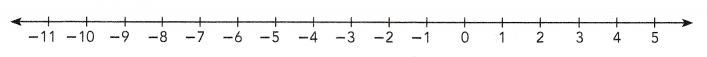Question 1.
|-10|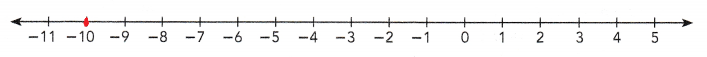The absolute value of the number |-10| is 10.
-10 is 10 units from 0.

Question 2.
|3|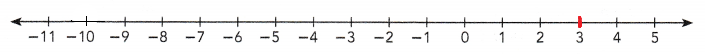The absolute value of the number |3| is 3.
3 is 3 units from 0.

Question 3.
|-8|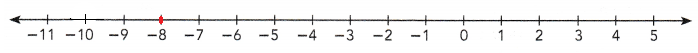The absolute value of the number |-8| is 8.
-8 is 8 units from 0.

Question 4.
|1|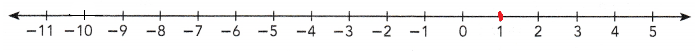The absolute value of the number |1| is 1.
1 is 1 units from 0.

Question 5.
|-7|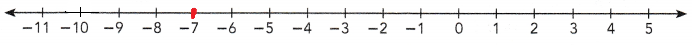The absolute value of the number |-7| is 7.
-7 is 7 units from 0.

Question 6.
|0|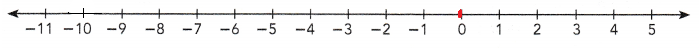The absolute value of the number |0| is 0.

Write the absolute value of each number.

Question 7.
|-23|
The absolute value of the number |-23| is 23.

Question 8.
|41|
The absolute value of the number |41| is 41.

Question 9.
|-38|
The absolute value of the number |-38| is 38.

Question 10.
|114|
The absolute value of the number |114| is 114.

Question 11.
|-132|
The absolute value of the number |-132| is 132.

Question 12.
|506|
The absolute value of the number |506| is 506.

Use absolute values to interpret real-world situations.

a) The figure shows a section from Keith’s bank account statement.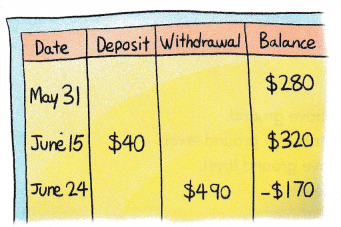As of May 31, Keith had $280 ¡n his bank account. On June 24, after he withdrew$490, he had -$170 in his bank account. |-170| = 170 This means that Keith had overdrawn$170.

b) A dog is standing on a cliff, 35 feet above sea level. A dolphin is swimming 6 feet below sea level. An octopus is moving along the seabed, 40 feet below sea level.
You can use positive and negative numbers to show the elevation of the animals relative to sea level.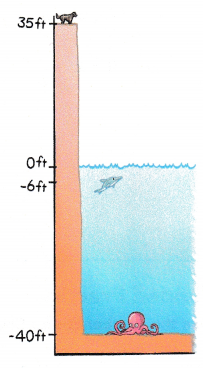Dog’s elevation = 35 ft
Dolphin’s elevation = -6 ft
Octopus’s elevation = -40 ft
To decide which animal ¡s farthest from sea level, you do not need to think about whether the animals are above or below sea level. You can use absolute values to compare distances.
Distance of dog from sea level = |35|
= 35 ft
Distance of dolphin from sea level = |-6|
= 6 ft
Distance of octopus from sea level = |-40|
= 40 ft
The octopus is farthest from sea level.

Question 13.
Joe owes his sister Lisa $12, and his sister Kelly$18. His brother David owes Joe $20. a) Joe writes the number -12 to represent the amount he owes Lisa. What numbers should Joe use to represent the other amounts given above? Answer: -18 to represent the amount he owes Kelly. 20 to represent the amount his brother David owes Joe. b) Which person owes the most money? Answer: Joe owes the most money($12 + $18 =$30)

Question 14.
At a parking garage, you can park underground or above ground. The lowest part of the underground parking is 40 feet below ground level. The highest part of the parking garage is 20 feet above ground level. The limousine parking area is 23 feet below ground level.
a) Use positive and negative numbers to represent the locations, with respect to ground level, of the three different parts of the parking
garage.
The lowest part of the underground parking is 40 feet below ground level which represents -40 feet.
The highest part of the parking garage is 20 feet above ground level which represents 20 feet.
The limousine parking area is 23 feet below ground level which represents -23 feet.

b) Which part of the parking garage is closest to ground level?
The highest part of the parking garage is 20 feet above ground level which is closest among all levels of parking.

### Math in Focus Course 1A Practice 2.2 Answer Key

Use the number line to find the absolute value of each of the following numbers.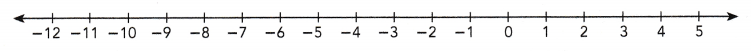Question 1.
|-11|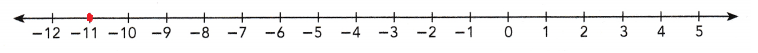The absolute value of the number |-11| is 11.
-11 is 11 units from 0.

Question 2.
|4|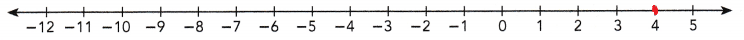The absolute value of the number |4| is 4.
4 is 4 units from 0.

Question 3.
|-6|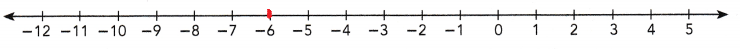The absolute value of the number |-6| is 8.
-6 is 6 units from 0.

Write the absolute value of each number.

Question 4.
|35|
The absolute value of the number |35| is 35.

Question 5.
|-46|
The absolute value of the number |-46| is 46.

Question 6.
|-77|
The absolute value of the number |-77| is 77.

Copy and complete each inequality using > or <.

Question 7.
|-26|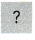|30|
|-26|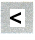|30|
Explanation:
The absolute value of the number |-26| is 26.
The absolute value of the number |30| is 30.
So, the number |-26| is less than |30|.

Question 8.
|-92||-114|
|-92|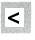|-114|
Explanation:
The absolute value of the number |-92| is 92.
The absolute value of the number |-114| is 114.
So, the number |-92| is less than |-114|.

Question 9.
|511||-500|
|511|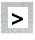|-500|
Explanation:
The absolute value of the number |511| is 511.
The absolute value of the number |-500| is 500.
So, the number |511| is greater than |-500|.

Question 10.
|-707||-628|
|-707|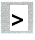|-628|
Explanation:
The absolute value of the number |-707| is 707.
The absolute value of the number |-628| is 628.
So, the number |-707| is greater than |-628|.

Question 11.
Two numbers have an absolute value of 16. Which of the two numbers is greater than 12?
The absolute value of -16 is 16 and the absolute value of 16 is 16. So we know that 16 is greater than 12 but -16 is not.

Question 12.
Math Journal Jesse graphed a point to represent the absolute value of a number on a number line. If the original number is less than -10, describe all the possible values for the point Jesse graphed on the number line. Explain
The possible values for the point Jesse graphed on the number line will be the values which are less than or equal to -11
Explanation:
Jessie pointed a number less than -10 on the number line. So the possible values will start from -11 and will decrease further.

Question 13.
The table shows a monthly bank account statement for the period March to July.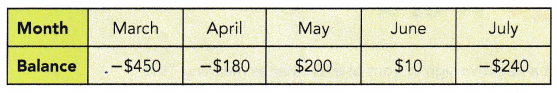a) For which months is the account overdrawn?
The account overdrawn in March, April and July months.

b) How much was the bank owed in March?
The bank owed $450 in March. c) In which month was the account overdrawn by the greatest amount? Answer: In March month the account overdrawn by the greatest amount with$450.

d) In which month was the account overdrawn by the least amount?
In April month the account overdrawn by the least amount with $180. e) How much was the bank owed ¡n total? Answer:$450 + $180 +$240 = $870 In total the bank owed is$870.

Question 14.
The table shows some locations with their elevations.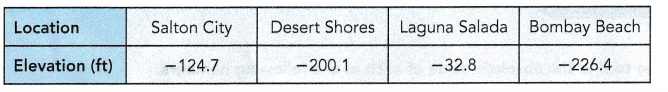a) Which location is the closest to sea level?
The location closest to sea level is Laguna Salada.

b) Which locations are within 200 feet of sea level?
The locations within 200 feet of sea level are Salton City and Laguna Salada.

c) How much farther from sea level is Desert Shores than Salton City?
-75.4 farther from sea level is Desert Shores than Salton City.

d) Write the locations ¡r, order from the location that is farthest from sea level to the location that is closest to sea level.
The location that is farthest from sea level to the location that is closest to sea level are Bombay Beach, Desert Shores, Salton City, Laguna Salada.

Question 15.
The table shows the average surface temperature of some planets.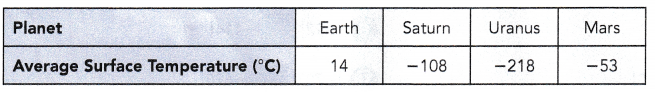a) Which planet has the highest average surface temperature?
Earth has the highest average surface temperature.

b) Which planet has the lowest average surface temperature?
Uranus has the lowest average surface temperature.

c) On Earth, the boiling temperature of water at sea level is 100°C. Which planet has an average surface temperature that is closest to this temperature?
Earth has an average surface temperature that is closet to the temperature 100°C.

d) Order the temperatures from lowest to highest.
The temperature from lowest to highest are -218, -108, -53, 14.

Brain @ Work

Question 1.
You can interpret a negative sign in front of a number as meaning “the opposite of.” So, -3 means the opposite of 3.

a) What number is -(-3) the opposite of?
The opposite number of -(-3) is -3.

b) What number is -(-3) equal to?